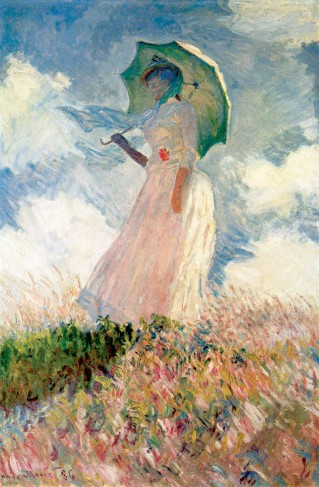# R - Colorspace (Manipulation)

Color Space Manipulation.

The package colorspace provides various functions providing perceptually-based color palettes for coding:

• categorical data (qualitative palettes)
• and numerical variables (sequential and diverging palettes).

The R package colorspace carries out mapping between assorted color spaces. Qualitative, sequential, and diverging color palettes based on HCL colors are provided.

## GUI

A graphical user interface for choosing colors based on the ideas of Zeileis et al. (2009) is provided in the package as choose_palette().

``````library("colorspace")
myPalette <- choose_palette()```
```

## Example

Convenience function for the examples

``````library("colorspace")
pal <- function(col, border = "light gray", ...)
{
n <- length(col)
plot(0, 0, type="n", xlim = c(0, 1), ylim = c(0, 1), axes = FALSE, xlab = "", ylab = "", ...)
rect(0:(n-1)/n, 0, 1:n/n, 1, col = col, border = border)
}```
```

### Qualitative Palette

``````pal(rainbow_hcl(4, start = 30, end = 300), main = "dynamic")
pal(rainbow_hcl(4, start = 60, end = 240), main = "harmonic")
pal(rainbow_hcl(4, start = 270, end = 150), main = "cold")
pal(rainbow_hcl(4, start = 90, end = -30), main = "warm")```
```

Examples for qualitative palettes. Hue is varied in different intervals for given C(hroma) = 50 and L(uminance) = 70.

## Documentation / Reference

Discover MoreColor - Palette

A palette is a finite number of color used in a design. theme type (color scheme)... Reference: - De...R - Package

R functionality is divided into a number of packages. The package contained in the “base” system include utils, stats, datasets, graphics, grDevices, grid, methods, tools, parallel, compiler, splines,...R - Plotly

R module of Plotly Installing 11 packages: colorspace, dichromat, ggplot2, gtable, labeling, munsell, plyr, proto, RColorBrewer, reshape2, scales Login parameter...